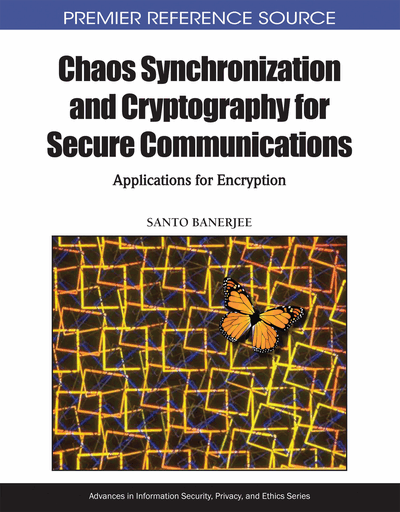# Simple Chaotic Electronic Circuits

M.P. Hanias (Technological and Educational Institute of Chalkis, Greece) and G. S. Tombras (University of Athens, Greece)
Copyright: © 2011 |Pages: 23
DOI: 10.4018/978-1-61520-737-4.ch004
OnDemand PDF Download:
Available
\$37.50
No Current Special Offers

## Abstract

Simple chaotic electronics circuits as diode resonator circuits, Resistor-Inductor-LED optoelectronic chaotic circuits, and Single Transistor chaotic circuits can be used as transmitters and receivers for chaotic cryptosystems. In these circuits we can change and investigate the influence of various circuit parameters to the complexity of the so generated strange attractors. Time series analysis is performed following Grassberger and Procaccia’s method while invariant parameters as correlation, and minimum embedding dimension are respectively calculated. The Kolmogorov entropy is also calculated and the RLT circuits in a critical state are examined.
Chapter Preview
Top

## 1. Introduction

In recent years, a growing number of cryptosystems based on chaos have been proposed. The majority of them are based on parameter modulation of a chaotic oscillator being the transmitter while the receiver is also a chaotic system synchronized by means of an adaptive observer. For example, a Lorenz’s attractor is used as the non-linear time-varying system while the encryption process modulates the parameters that affect the shape and complexity of the corresponding strange attractor with the binary encoded plaintext. In general, the security of chaotic cryptosystems is based on the chaotic behaviour of the output of electronic non-linear systems which produce Lorenz type time series. In order to enhance the level of security of the transmission system various novel communication schemes that combine conventional cryptographic methods and synchronization of chaotic systems have been developed. A common feature of these systems is the utilization of the state variables of the chaotic systems (other than the transmitted state) as encryption keys and decryption algorithms. In such a system, a low-dimensional Chua’s circuit as a chaotic system is used as an independent low-dimensional chaotic system that sends a driving signal to two chaotic slave systems, which are then synchronized and used to generate confidential keys.

A good example of a chaotic communication system is the Tsu-I Chien and Teh–Lu Liao system, the block diagram of which is shown in Figure 1. As it can be readily seen, the complete system comprises four modules, namely the Chaotic Modulator (CM), the Chaotic Secure Transmitter (CST), the Chaotic Secure Receiver (CSR), and the Chaotic Demodulator (CDM).

In the CM, an off-line self-learning process is executed prior to the transmitting period. During this learning process, a novel modulation scheme is applied to establish analog chaotic patterns corresponding to the input bit sets. Subsequently, certain parameters of these chaotic patterns (i.e. the initial peak values) are digitized and stored in the modulator’s memory. In the transmitting period, a D/A converter is utilized to retrieve the digitalized parameters corresponding to the input bits from the modulator’s memory. These parameters are then used to perturb the chaotic circuit (a Rossler-like system) so that analog chaotic patterns, as opposed to the original input bits, can be transmitted to the CST.

The CST and CSR modules are designed in such a way that a single scalar signal is transmitted across the public channel. By giving certain structural conditions as such of a particular class of chaotic systems (e.g. Chua’s circuits), the main transmitter and the nonlinear observer-based main receiver (with an appropriate observer gain) are constructed to synchronize with each other. These two slave systems are driven simultaneously by the transmitted signal and are designed to synchronize and generate confidential keys. Synchronization between the chaotic circuits in the CST and CSR modules is guaranteed through the Lyapunov stability theorem.

The output of the CSR is then supplied to the CDM. The chaotic circuit of this module has the same system parameters as those of the CM, but does not insist on the same initial states as those in the latter, since the demodulator and the modulator can only be synchronized after a short transient period by using the proposed nonlinear observer-based synchronization scheme. In the CDM, a bit detector measures the output of the CDM’ s chaotic circuit and matches it to the corresponding bit set, thereby recovering the transmitted input bits. Hence, it becomes clear that control and synchronization of the proposed system operation is based on analog circuits such as Chua’s circuits and Rossler-like systems, i.e. on simple and easily controlled analogue circuits that can readily reveal chaotic operation with low dimensionality.

## Complete Chapter List

Search this Book:
Reset